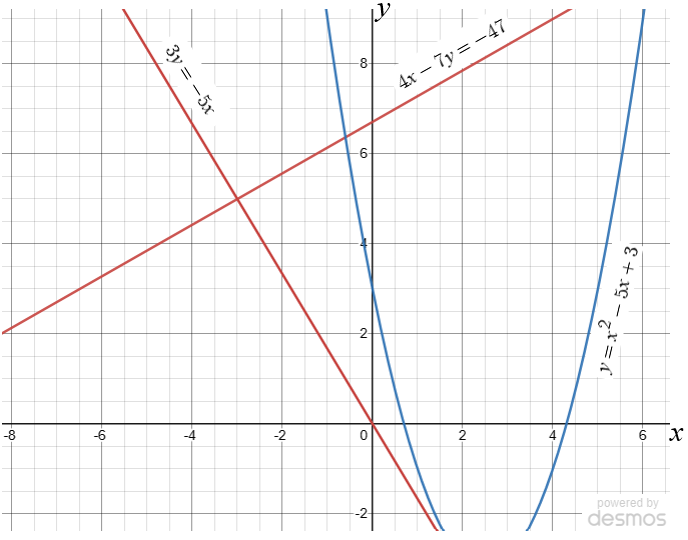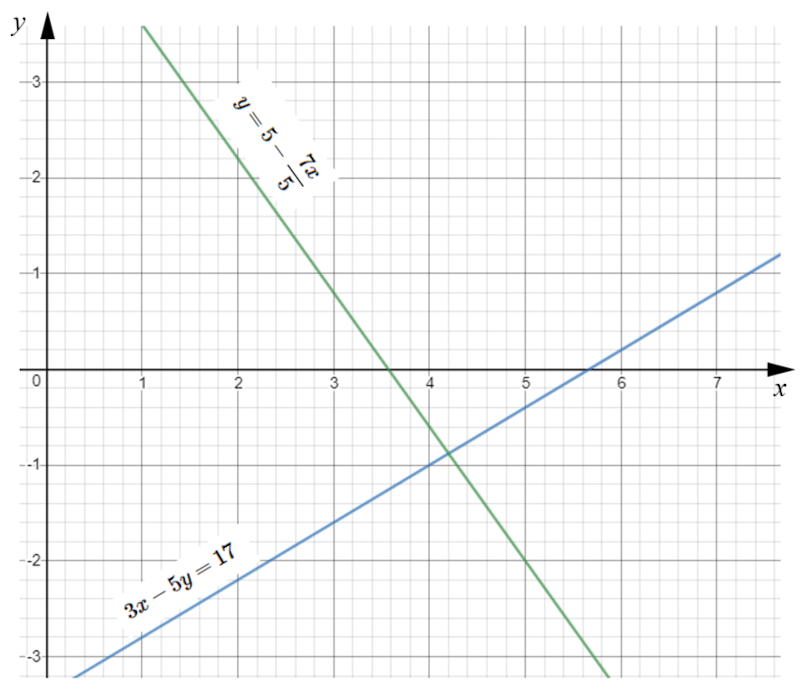# Exam-Style Questions.

## Problems adapted from questions set for previous Mathematics exams.

### 1.

GCSE Higher

(a) Use the red graphs to solve the simultaneous equations:

$$4x-7y=-47$$ $$3y=-5x$$

(b) Use the blue graph to find estimates for the solutions of the quadratic equation:

$$x^2-5x+3=0$$### 2.

GCSE Higher

Estimate the solutions of the following simultaneous equations using their graphs as drawn on the grid below.

$$3x-5y=17$$ $$y=5-\frac{7x}{5}$$If you would like space on the right of the question to write out the solution try this Thinning Feature. It will collapse the text into the left half of your screen but large diagrams will remain unchanged.

The exam-style questions appearing on this site are based on those set in previous examinations (or sample assessment papers for future examinations) by the major examination boards. The wording, diagrams and figures used in these questions have been changed from the originals so that students can have fresh, relevant problem solving practice even if they have previously worked through the related exam paper.

The solutions to the questions on this website are only available to those who have a Transum Subscription.

Exam-Style Questions Main Page

Search for exam-style questions containing a particular word or phrase:

To search the entire Transum website use the search box in the grey area below.